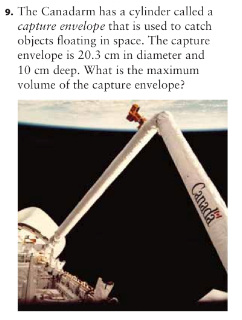## Tuesday, March 1, 2011

### Carl's Volume Scribe Post

Cylinder Volume and Volume problems

Here are the following examples of questions that I answered :

Section 7.3 : Pages 265-267* height = 10cm

* diameter = 20.3cm
r = d/2
r = 20.3/2
r = 10.15cm

v = π x r x r x h
v = 3.14 x 10.15 x 10.15
v = 323.49cm2
v = 323.49 x 10

Final Answer : v = 3234.9cm3

Section 7.4 : Pages 272-275

* height = 10m
* diameter = 0.8 (inside) and 1 (outside)

Inside :
r = d/2
r = 0.8/2
r = 0.4

v = π x r x r x h
v = 3.14 x 0.4 x 0.4 x h
v = 0.5 x 10
v = 5m

Outside :
r = d/2
r = 1/2
r = 0.5

v = π x r x r x h
v = 3.14 x 0.5 x 0.5 x h
v = 0.78 x 10
v = 7.8m

We are trying to find a way to find the volume of the concrete needed to make the culvert. So what I did is that I took the total from the volume of the inside concrete and just subtracted from the total of the outside concrete.

Inside = 5m
Outside = 7.8m

What I did :
7.8 - 5m = 2.8m3

The concrete required to make the culvert is 2.8m3

#### 1 comment:

1.Great Job Carl! I like how you put different colours to say that it is important and your work is very neat and understandable. Keep it up !